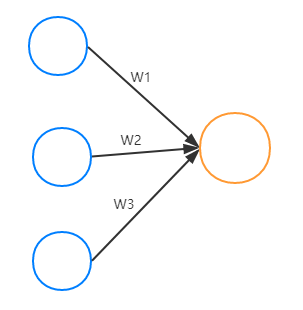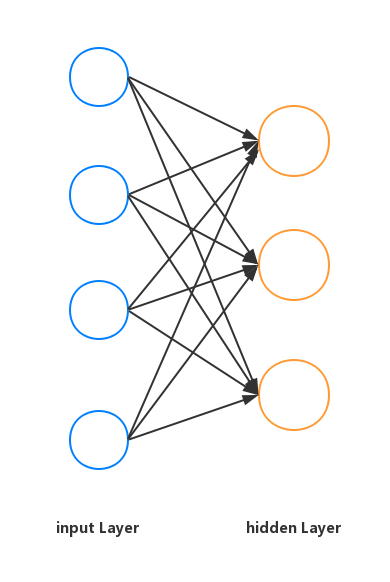# 神经网络关于输入X 权重W 和偏置biase 的Shape形式辨析

logistics 回归中权重的表述${f}_{ac}\left({\theta }^{T}\cdot X+b\right),\phantom{\rule{1em}{0ex}}{f}_{ac}:activationfunction$fac(WX+b),fac:activationfunction f a c ( W ⋅ X + b ) , f a c : a c t i v a t i o n f u n c t i o n

W3×4X4×10matrix3×10 W 3 × 4 ⋅ X 4 × 10 → m a t r i x 3 × 10

w11w21w31w12w22w32w13w23w33w14w24w34x(1)1x(1)2x(1)3x(1)4x(2)1x(2)2x(2)3x(2)4............x(10)1x(10)2x(10)3x(10)4+b1b2b3 | w 11 w 12 w 13 w 14 w 21 w 22 w 23 w 24 w 31 w 32 w 33 w 34 | ⋅ | x 1 ( 1 ) x 1 ( 2 ) . . . x 1 ( 10 ) x 2 ( 1 ) x 2 ( 2 ) . . . x 2 ( 10 ) x 3 ( 1 ) x 3 ( 2 ) . . . x 3 ( 10 ) x 4 ( 1 ) x 4 ( 2 ) . . . x 4 ( 10 ) | + | b 1 b 2 b 3 |

# -*- coding: utf-8 -*-
"""
Created on Mon Mar 19 15:24:06 2018

@author: lyh
"""

import tensorflow as tf
from tensorflow.examples.tutorials.mnist import input_data

W = tf.Variable(tf.random_normal([in_size,out_size]),name="wight")
b = tf.Variable(tf.zeros([1,out_size]) + 0.1,name="biase")
if activation_function is None:
ouput = tf.matmul(inputs,W) + b
else:
ouput = activation_function(tf.matmul(inputs,W) + b)
return ouput

def compute_accuracy(v_xs,v_ys):
global prediction
y_pre = sess.run(prediction,feed_dict={xs:v_xs})
correct_prediction = tf.equal(tf.argmax(y_pre,1),tf.argmax(v_ys,1))
accuracy = tf.reduce_mean(tf.cast(correct_prediction,tf.float32))
result = sess.run(accuracy,feed_dict={xs:v_xs,ys:v_ys})
return result

xs = tf.placeholder(tf.float32,[None,784]) #这里是28*28的照片，列数是784为特征维度
ys = tf.placeholder(tf.float32,[None,10])  #列数是10为输入的维度
#两个None 分别代表实际输入样本的个数

cross_entropy = tf.reduce_mean(-tf.reduce_sum(ys * tf.log(prediction),reduction_indices=)) #loss

#初始化
init = tf.initialize_all_variables()
sess = tf.Session()
sess.run(init)

for step in range(1000):
batch_xs,batch_ys = mnist.train.next_batch(100)
sess.run(train,feed_dict={xs:batch_xs,ys:batch_ys})
if step % 50 == 0:
print(compute_accuracy(mnist.test.images,mnist.test.labels))

05-051834
08-11474404-254万+
04-24
04-092万+
12-271万+
09-031万+
03-293449
09-15